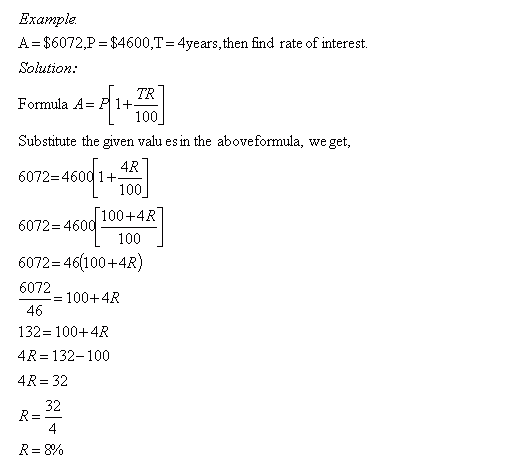Name: ___________________Date:___________________

 Email us to get an instant 20% discount on highly effective K-12 Math & English kwizNET Programs!

Grade 8 - Mathematics9.32 Simple Interest - VIIIDirections: Solve the following problems. Also write at least ten examples of your own.
 Q 1: A = \$1725, P = \$1500 and T = 3years, then find rate of interest.2.5%2%5%6% Q 2: A = \$3556, P = \$2800 and T = 3years, then find rate of interest.8%6%9%3% Q 3: A = \$10230, P = \$9300 and T = 3years, then find rate of interest.2.5%2%1%1.5% Q 4: A = \$5428, P = \$4600 and T = 2years, then find rate of interest.6%9%5%8% Q 5: A = \$16352, P = \$14600 and T = 6years, then find rate of interest.1.5%3%2%4.5% Q 6: A = \$8284, P = \$7600 and T = 3years, then find rate of interest.2.5%3.5%3%4% Question 7: This question is available to subscribers only! Question 8: This question is available to subscribers only!